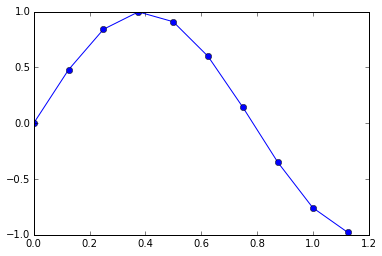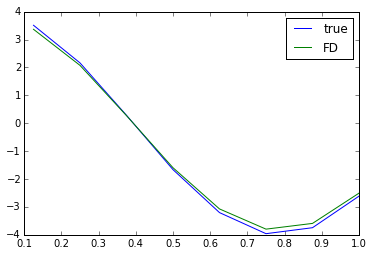# Finite Differences

In :
import numpy as np
import numpy.linalg as la
import matplotlib.pyplot as pt


### Part 1: Examining the Differentiation Matrix

In :
degree = 2
h = 0.25

# Assume even degree so that there's a well-defined middle node.
assert degree % 2 == 0

nodes = np.linspace(-h/2, h/2, degree+1)
nodes

Out:
array([-0.125,  0.   ,  0.125])


Now construct V (the generalized Vandermonde) and Vprime (the generalized Vandermonde for the derivatives):

In :
V = np.array([
nodes**i
for i in range(degree+1)
]).T

In :
def monomial_deriv(i, x):
if i == 0:
return 0*x
else:
return i*nodes**(i-1)

Vprime = np.array([
monomial_deriv(i, nodes)
for i in range(degree+1)
]).T


Combine them to form the derivative matrix:

In :
diff_mat = Vprime.dot(la.inv(V))
diff_mat

Out:
array([[-12.,  16.,  -4.],
[ -4.,   0.,   4.],
[  4., -16.,  12.]])


Let's say we only care about the derivative at the middle node:

In :
finite_difference_weights = diff_mat[degree//2]
finite_difference_weights

Out:
array([-4.,  0.,  4.])

• What have we learned?
• What formula does this amount to?
• How do these weights change if we change $h$?
• What formula does this amount to, really?
• What happens if we change the degree?
• What happens if we shift all nodes?
In :
# * We could have left the middle point out. :)
# * -4*f(x-0.25) + 4*f(x+0.25)
# * They scale with 1/h, as you might expect.
# * (f(x-h/2) + f(x+h/2))/h
# * We get a more complicated (but more accurate) formula (with more source nodes)
# * The weights remain the same.


### Part 2: Using finite difference formulas

In :
def f(x):
return np.sin(4*x)
def df(x):
return 4*np.cos(4*x)

In :
x = np.arange(10) * 0.125
pt.plot(x, f(x), "o-")

Out:
[<matplotlib.lines.Line2D at 0x7f761043a198>]Now use the weights to compute the finite difference derivative as deriv:

In :
fdw = finite_difference_weights

fx = f(x)
deriv = np.zeros(len(x)-2)
for i in range(1, 1+len(deriv)):
deriv[i-1] = fx[i-1]*fdw + fx[i]*fdw + fx[i+1]*fdw


Now plot the finite difference derivative:

In :
pt.plot(x[1:-1], df(x[1:-1]), label="true")
pt.plot(x[1:-1], deriv, label="FD")
pt.legend()

Out:
<matplotlib.legend.Legend at 0x7f7610378860>